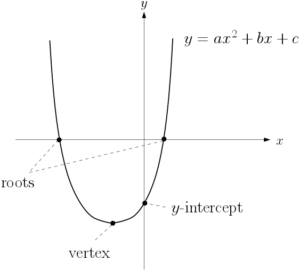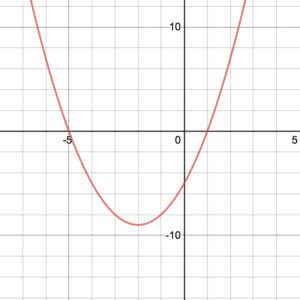A quadratic expression is any expression with an x squared term, an x term and a constant. For example, $3x^2-4x+7$ is a quadratic expression. Note that it doesn’t have to be an $x$, it could by $y$ or any other letter as long as it is the same throughout. Furthermore, there are three ways in which you can solve quadratics – each method requires setting the quadratic to 0 first. See Example 1.

### Factorising

Firstly, the simplest method, provided that it is possible, is factorising.

Example: $2x^2-5x-3=0\hspace{5pt}\Rightarrow\hspace{5pt}(2x+1)(x-3)=0\hspace{5pt}\Rightarrow\hspace{5pt} x=-\frac{1}{2}, x=3$.

If factorising doesn’t work but a quadratic does have roots, the quadratic formula will find them instead. Recall that the discriminant will tell you how many roots a quadratic has.  See Discriminants page.
The quadratic formula says that if $ax^2+bx+c=0$ then the roots are given by:

$x=\frac{-b\pm\sqrt{b^2-4ac}}{2a}$

Example: In the following quadratic, $a=1$, $b=3$ and $c=-3$:

$x^2+3x-3=0\hspace{5pt}\Rightarrow\hspace{5pt}x=\frac{-3\pm\sqrt{3^2-4\times 1\times-3}}{2}\hspace{5pt}\Rightarrow\hspace{5pt}x=\frac{-3 +\sqrt{21}}{2}, \frac{-3-\sqrt{21}}{2}$

given exactly, i.e. not as a rounded decimal. Rounded to two decimal places using a calculator, the solutions are $x=0.79$ and $x=-3.79$. See Example 2.

### Completing the Square

Alternatively, another infallible method for finding roots (if a quadratic is solvable) is to complete the square. See Completing the Square page and Example 3.

Example: $x^2+6x+5=0\hspace{3pt}\hspace{5pt}\Rightarrow\hspace{5pt}(x+3)^2-4=0\hspace{5pt}\Rightarrow\hspace{5pt} x+3=\pm 2\hspace{3pt}\hspace{5pt}\Rightarrow\hspace{5pt} x=-1, x=-5$

It is worth noting that completing the square is also useful for sketching a quadratic. The reason for this is that, by writing the quadratic in completed square form, we can see the transformations applied to the graph of $x^2$ (the shape of a quadratic is a known as a parabola). For example, to get $y=(x+3)^2+1$ we shift the graph of $x^2$ by 3 ($x$ transformation) and then up by 1 ($y$ transformation).1. Firstly, find the roots using one of the above methods, roots occur when $y=0$.

2.  Then, find the $y$-intercept, this occurs when $x=0$.

3. Finally, find the coordinates of the vertex by completing the square and applying transformations to $y=x^2$.

See Completing the Square for more details and check out Example 4.DESMOS is a fantastic sketching tool. Click here to try it out. Firstly click the start graphing button and type y=x^2+4x-5 in the bar where the cursor starts. Then try adding more graphs and experimenting with the options. Finally, try exporting your graphs.

Solve the following:

1. $x^2+x=12$
2. $2x^2=12x-16$

Solve $2x^2-5x-6=0$, giving your answers to two decimal places.

Solve the equation $2x^2+6x-9=0$ by completing the square. Give exact answers, simplified where appropriate.